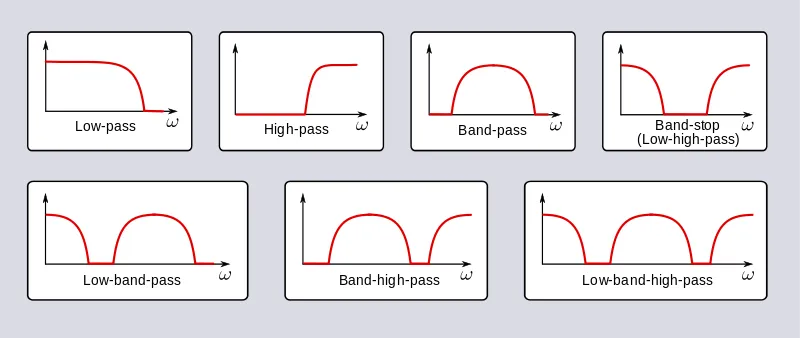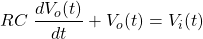# Mathematics - Signals And Systems - Filtering[Image1]

## Introduction

Hey it's a me again @drifter1!

Today we continue with my mathematics series about Signals and Systems in order to cover Filtering.

So, without further ado, let's dive straight into it!

## Convolution Property

Before we get into Filtering, its wise to recall the convolution property of the Fourier transforms, as this property is the foundation Filtering is built upon. In either Time-Domain, the convolution of two signals turns into multiplication in their Fourier Transforms:In the exercises about LTI systems, this property helped us simplify the calculations for the system response/output. Moving into the Frequency-Domain turned all the complicated convolution calculations into simple multiplications of known Fourier Transforms. To get even more specific, in LTI systems the Fourier transform of the output is equal to the Fourier transform of the input multiplied by the frequency response, which in turn is simply the Fourier transform of the impulse response.

## Getting into Filtering

So, how does the convolution property of the Fourier transform lead to Filtering? Well, multiplying the Fourier transform of the input, which is a "frequency representation" of the signal, by the frequency response "shapes" the input in some manner. Depending on the frequency response, only some frequency bands are "allowed" to pass, whilst others are rejected. As such, the frequency response acts as a frequency-selective filter.

## Ideal Filters

The Ideal frequency-selective filters are:

• Low-pass
• High-pass
• Band-pass
• Band-reject
They are useful mathematical abstractions, which are not implementable.

Non-exact design filters have a less severe transition from the passband to the stopband, which is preferable and more suitable in practical situations. Consequently a transition region is introduced between the two main frequency-bands. Graphically, such filters give results, that look like this:[Image 2]

## Series R-C Filter Approximation

A simple approximation of a frequency-selective filter is the series RC circuit. The output taken across the capacitor "rejects" high frequencies and can be used as an approximation of a low-pass filter. On the other hand, the output across the resistor "rejects" low frequencies, and as such approximates high-pass filters.

A simple series R-C circuit is of the following form:[Image 3]

Using Kirchhoff's Laws its possible to define the circuit mathematically as:Using the Fourier transform results in:As such, considering the voltage across the capacitor, Vo(ω), the low-pass filter can be described as:Considering the voltage across the resistor, VR(ω), gives us the high-pass filter:## Moving Average Filter Approximation

In the case of Discrete-Time, moving average filters tend to be used. Such filters have finite-length impulse response and average together adjacent values as they move through them. This procedure is commonly used in the stock market to smooth out the day-to-day fluctuations and in turn to represent long-time trends. In filters, this means retaining lower frequencies and rejecting (smoothing out) high frequencies.

Mathematically, moving average filters looks like this:Recursive moving average filters are also commonly used for discrete-time filters. A simple first-order difference equation can easily approximate such a low-pass or high-pass filter:Using the Fourier transform and its various properties leads us to:The final result is the Fourier transform of h[n] = an u[n].

## RESOURCES:

### Images

1.2.3.Block diagrams and other visualizations were made using draw.io and GeoGebra

## Final words | Next up

And this is actually it for today's post!

Next time we will get into Modulation...

See Ya!Keep on drifting!

H2
H3
H4
3 columns
2 columns
1 column
Join the conversation now